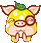登录 注册# 技术帝―对于天籁梵音技能的研究（附图）

 关键词：天籁梵音、强克、平民仙、杂克、不变卡、计算杂克伤害   一、基础数据 >>推荐阅读：另类观点——贫民大仙对五行技能的分析       二、结论    1、定义    设定：X1=克金数值、X2=克木数值、X3=克水数值、X4=克火数值、51=克土数值   设定：S=总伤害、S1=平秒伤害   2、结论   （1）不变卡、杂克用天籁梵音技能   强克总伤害S=S1*(1+X1*50%)*(1+X2*50%)(1+X3*50%)(1+X4*50%)(1+X5*50%)   数据支撑：   34179=24607*（1+43.1%*50%）*（1+9.5%*50%）*（1+9.3%*50%）*（1+0%*50%）*（1+8.5%*50%）   22786=16405*（1+43.1%*50%）*（1+9.5%*50%）*（1+9.3%*50%）*（1+0%*50%）*（1+8.5%*50%）   （2）不变卡、用饮金技能   强克总伤害S=S1*(1+X1*50%)   数据支撑：29909=24607*（1+43.1%*50%）   19940=16405*（1+43.1%*50%）   （3）对于男鬼而言，三尸伤害强克有效   强克总伤害S=S1*(1+X1*50%)*(1+X2*50%)*(1+X3*50%)*(1+X4*50%)*(1+X5*50%)   数据支撑：7633=7161*(1+13.2*50%)*(1+0*50%)*(1+0*50%)*(1+0*50%)*(1+0*50%)   （4）对于平民而言，在杂克总点数一定的情况下，尽量平均分布杂克。   比如杂克100，那么平均分布强克，克金木水火土各克20,可以达到最佳杀伤效果，相比单克100不变卡的伤害提高7.3%的伤害，原因如下：   （1+20%*50%)*（1+20%*50%)*（1+20%*50%)*（1+20%*50%)（1+20%*50%)=(1+100%*50%)*1.073   同理，如杂克70，那么平均分布强克，克金木水火土各克14,可以达到最佳杀伤效果，相比单克70不变卡的伤害提高7.3%的伤害3.8%，自己推导！   以上内容为玩家原创，不代表叶子猪观点，具体内容还请大家在游戏中尝试才知哦！ <<<点击进入大话西游2论坛讨论>>> [编辑：韦小贝]